How Cheenta works to ensure student success?
Explore the Back-Story

# Test of Mathematics Solution Subjective 43-Integer RootThis is a Test of Mathematics Solution Subjective 43 (from ISI Entrance). The book, Test of Mathematics at 10+2 Level is Published by East West Press. This problem book is indispensable for the preparation of I.S.I. B.Stat and B.Math Entrance.

Also visit: I.S.I. & C.M.I. Entrance Course of Cheenta

## Problem

Show that the equation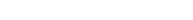has no integral root for any integer n.

## Solution:

We note that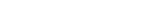implies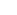is divisible by 7. This implies x is divisible by 7 (as 7 is a prime number). Suppose x= 7x'. Hence we can rewrite the given equation as: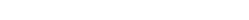.

Cancelling out a 7 we have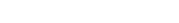. Since 7 divides left hand side, it must also divide the right hand side. Since 7 cannot divide 2, it must divide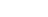as 7 and 2 are coprime.  Note that 7 cannot divideas square of a number always gives remainder 0, 1, 4, 2 when divided by 7 and never 6. But ifis divisible by 7 then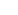must give remainder 6 when divided by 7.  Hence contradiction.

Necessary Lemma: square of a number always gives remainder 0, 1, 4, 2 when divided by 7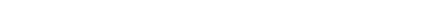Key Ideas: Modular ArithmeticThis is a Test of Mathematics Solution Subjective 43 (from ISI Entrance). The book, Test of Mathematics at 10+2 Level is Published by East West Press. This problem book is indispensable for the preparation of I.S.I. B.Stat and B.Math Entrance.

Also visit: I.S.I. & C.M.I. Entrance Course of Cheenta

## Problem

Show that the equationhas no integral root for any integer n.

## Solution:

We note thatimpliesis divisible by 7. This implies x is divisible by 7 (as 7 is a prime number). Suppose x= 7x'. Hence we can rewrite the given equation as:.

Cancelling out a 7 we have. Since 7 divides left hand side, it must also divide the right hand side. Since 7 cannot divide 2, it must divideas 7 and 2 are coprime.  Note that 7 cannot divideas square of a number always gives remainder 0, 1, 4, 2 when divided by 7 and never 6. But ifis divisible by 7 thenmust give remainder 6 when divided by 7.  Hence contradiction.

Necessary Lemma: square of a number always gives remainder 0, 1, 4, 2 when divided by 7Key Ideas: Modular Arithmetic

### Knowledge Partner